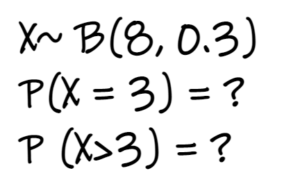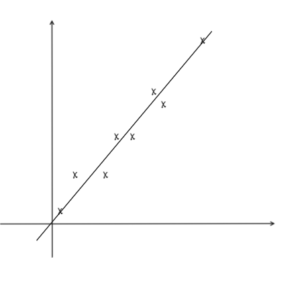# Category: Math

Here, you will find resources on O Level and A Level Mathematics resources.

## 2023 Mathematics Specimen Paper 2

In this post, you’ll find the worked solutions for the 2023 Mathematics specimen paper 2. 2023 will be the first year for syllabus 4052.

## 2023 Mathematics Specimen Paper 1

In this post, you’ll find the worked solutions for the 2023 Mathematics specimen paper 1. This will be the first year for syllabus 4052.## How to find probabilities for binomial distribution with TI 84 graphic calculator

Let’s look at how to find probabilities for binomial distribution with Ti 84 CE plus graphic calculator, tested in statistics section.## Sampling Questions: A Level TYS Answers

Find the worked solutions for past A Level sampling questions, which are found in paper 2 of H2 Math papers, statistics section.## Hypothesis Testing Questions: A Level TYS Answers

Find the worked solutions for past A Level hypothesis testing questions, which are found in paper 2 of H2 Math papers, statistics section.## Regression and Correlation Questions: A Level TYS Answers

Find the worked solutions for past A Level regression and correlation questions, found in paper 2 of H2 Math papers, statistics section.## Binomial Distribution Questions: A Level TYS Answers

Find the worked solutions for past A Level binomial distribution questions. Binomial distribution questions are found in paper 2, statistics section of A Level Math.## Normal Distribution Questions: A Level TYS Answers

Find the worked solutions for past A Level normal distribution questions. Normal distribution questions are found in paper 2, statistics section.## Discrete Random Variable Questions: A Level TYS Answers

Find the worked solutions for past A Level discrete random variable questions, which are found in paper 2 of H2 Math papers, statistics section.## Probability Questions: A Level TYS Answers

Find the worked solutions for past A Level probability questions. Probability questions are found in paper 2.